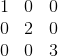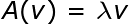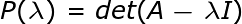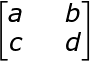# Diagonalization: Definition & Example

Instructor: Paul Bohan-Broderick

Paul has been teaching many subjects in many different ways since he received his PhD in 2001.

Diagonalization is the process of transforming a matrix into diagonal form. Diagonal matrices represent the eigenvalues of a matrix in a clear manner. This lesson will focus on finding the diagonalized form of a simple matrix.

## Diagonalization

A diagonal matrix is a matrix in which non-zero values appear only on its main diagonal. In other words, every entry not on the diagonal is 0. Diagonalization is the process of transforming a matrix into diagonal form.Not all matrices can be diagonalized. A diagonalizable matrix could be transformed into a diagonal form through a series of basic operations (multiplication, division, transposition and so on). However, this process can be long and is not easily described. Fortunately, diagonalization can be carried out through a more general algorithm that takes advantage of the matrix's characteristic polynomial.

## Eigenvectors and Eigenvalues

Since eigenvectors and eigenvalues of a matrix are so important for understanding the how and why of a diagonal matrix, it would be worthwhile to quickly review them here. A matrix, say A, can be understood to represent a function or transformation that could be applied to a vector, say v. If the vector changes in magnitude, but does not otherwise transform, then it is an eigenvector of the matrix. Whatever value the vector is changed by is called the eigenvalue of the matrix. Usually the scalar number by which the vector is multiplied is called lambda. This relationship can be expressed through the following equation:The diagonalized matrix is not in the same vector space as the original matrix. The eigenvectors of the matrix will be the basis of the new space. When a matrix has been diagonalized, the columns of each corresponds to an eigenvector of the matrix and each value (one per column) represent the eigenvalues of the matrix.

## Characterstic Polyniomial

Matrix diagonalization is usually carried out by manipulating the characteristic polynomial of the matrix. The eigenvalues of the matrix are the zeros of the characteristic polynomial.## Condition for Diagonalization

Not every matrix can be diagonalized.

Every square matrix has a characteristic equation. However, the values of the diagonalized matrix are the values of lambda when P(lambda) = 0. If the determinant of the characteristic function is not 0 for some lambda, then the matrix cannot be diagonalized.

## Description of the Diagonalization Process

The determinant of the square matrix is a useful property. The algorithm for computing the determinant gets more complicated as the matrix gets larger. For instance, the determinant of the 2x2 matrixaeibfgcdhcegbdiafh

Larger matrices require longer and more complicated computations.

The inner expression of the determinant equation is a new matrix created by multiplying the identity matrix by lambda and subtracting the original matrix by lambda. Taking the determinant of this new matrix will yield a polynomial. The zeros of that polynomial are the entries of the diagonal matrix. In effect, this new matrix is created by subtracting lambda from each entry on the diagonal of the matrix.

To unlock this lesson you must be a Study.com Member.

### Register to view this lesson

Are you a student or a teacher?

### Unlock Your Education

#### See for yourself why 30 million people use Study.com

##### Become a Study.com member and start learning now.
Back
What teachers are saying about Study.com

### Earning College Credit

Did you know… We have over 160 college courses that prepare you to earn credit by exam that is accepted by over 1,500 colleges and universities. You can test out of the first two years of college and save thousands off your degree. Anyone can earn credit-by-exam regardless of age or education level.